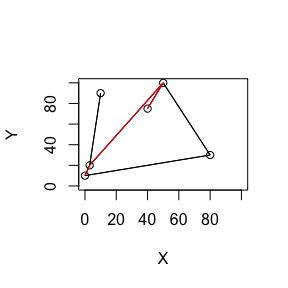# Application and use of package “locationgamer”

The package “locationgamer” identifies Nash Equilibrium locations in connected and disconnected networks. When using the package, the user only has to provide a matrix specifying which vertexes are connected, the x-y-coordinates of each vertex in the network, and a vector specifying demand/ revenue at each vertex.

The following sections discuss how a location game is defined in the context of this package, how one can identify a Nash Equilibrium location, and what the limitations of the current version are.

## How is a location game defined in the context of “locationgamer”?

I define a location game as the following scenario in the context of this package:

• A vertex constitutes a city, or town.
• Two competitors have to choose one location each for their service station (for example: ice-cream parlor, hotel, restaurant, gas station, etc.). Only a vertex can be chosen as a location.
• They need to choose the location simultaneously, i.e. without observing the other party’s choice.
• The competitors know each other’s payoff for each choice.
• The service provided by the competitors is so similar that customers choose the service locations closest to them.
• The network can be connected or disconnected. If the network is disconnected, customers in one part of the network cannot reach a service station located in the disconnected part.
• If a vertex is equidistant from two service stations, the respective demand/ revenue of the vertex is equally divided between the stations.

## What questions need to be answered to find a Nash Equilibrium location?

Before one can answer the question whether a location is a Nash Equilibrium location, one has to find the shortest path through a network. The shortest path is necessary to answer whether a customer goes to one service station or the other.

To find the shortest path, this package implements Dijkstra’s algorithm. To showcase the applicability of the package, we first create a network consisting of six nodes, which are distributed over the x-y-plane. The edges highlighted in red constitute the shortest path between vertex one and vertex six.

nNodes <- 6
xMin <- 0
xMax <- 100
yMin <- 0
yMax <- 100
coordMatrix <- matrix(c(0,10,3,20,10,90,80,30,50,100,40,75), nrow = nNodes, ncol =2, byrow = TRUE)
edgeMatrix <- matrix(0, ncol = nNodes, nrow = nNodes)
edgeMatrix[,1] <- c(0,1,0,1,0,0)
edgeMatrix[,2] <- c(1,0,1,0,1,0)
edgeMatrix[,3] <- c(0,1,0,0,0,0)
edgeMatrix[,4] <- c(1,0,0,0,1,0)
edgeMatrix[,5] <- c(0,1,0,1,0,1)
edgeMatrix[,6] <- c(0,0,0,0,1,0)

locationgamer::plotNetwork(edgeMatrix, coordMatrix)

startNode <- 1
endNode <- 6

dijkstraResult <- locationgamer::dijkstra(edgeMatrix, coordMatrix, startNode, endNode, nNodes)
locationgamer::plotDijkstra(edgeMatrix, coordMatrix, dijkstraResult[])Once the network is specified by its edges and vertex locations, one has to specify the demand/ revenue that can be generated in each location. Using the network above, we need to specify a vector of length six. For example, one can assume that demand at vertex 1 is 100, 300 at vertex 2, 50 at vertex 3, 400 at vertex 4, 200 at vertex 5, and 350 at vertex 6.

demandLoc <- c(100,300,50,400,200,350)

The function “lgsolve” then first determines the distance between all vertexes of the network, and iterates over all possible locations the two competitors can choose. It then divides the demand between the two competitors in the following way:

• If the competitors are equidistant to a location, the demand/ revenue at the respective location is divided evenly between the two competitors.
• The demand of a location that is closer to a competitor location, will be assigned to the competitor.
• In case the network is disconnected, a competitor can only generate demand/ revenue from the part of the network he or she is located in.

Each competitor then determines the location with the best demand/ payoff contingent on the other party’s choice, without actually observing the choice. If the choice of a particular location overlaps, it is a Nash Equilibrium location, which means that no party stands to gain from deviating from this location.

locationgamer::lgsolve(edgeMatrix, coordMatrix, 2, demandLoc)
#> $Equilibrium Locations #> [,1] [,2] [,3] [,4] [,5] [,6] #> [1,] 0 0 0 0 0 0 #> [2,] 0 0 0 0 0 0 #> [3,] 0 0 0 0 0 0 #> [4,] 0 0 0 0 0 0 #> [5,] 0 0 0 0 1 0 #> [6,] 0 0 0 0 0 0 #> #>$Equilibrium Summary
#>      Location_P1 Location_P2 Payoff_P1 Payoff_P2
#> [1,]           5           5       700       700

The function “lgsolve” then outputs all possible Nash Equilibrium locations, including the payoffs for the two competitors.

## Limitations of package “locationgamer”

The current limitations of this version of “locationgamer” are the following:

• Only supports two competitors
• Only supports strategic from games, in which competitors have to decide simultaneously
• Assumes that competitors know each other’s reward function
• Assumes only two locations will be chosen, one for each competitor

These limitations will be addressed in future releases of the package.